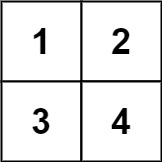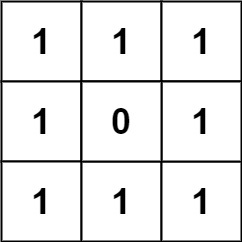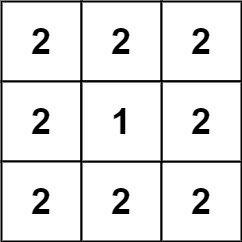## Algorithm

Problem Name: 892. Surface Area of 3D Shapes

You are given an `n x n` `grid` where you have placed some `1 x 1 x 1` cubes. Each value `v = grid[i][j]` represents a tower of `v` cubes placed on top of cell `(i, j)`.

After placing these cubes, you have decided to glue any directly adjacent cubes to each other, forming several irregular 3D shapes.

Return the total surface area of the resulting shapes.

Note: The bottom face of each shape counts toward its surface area.

Example 1:```Input: grid = [[1,2],[3,4]]
Output: 34
```

Example 2:```Input: grid = [[1,1,1],[1,0,1],[1,1,1]]
Output: 32
```

Example 3:```Input: grid = [[2,2,2],[2,1,2],[2,2,2]]
Output: 46
```

Constraints:

• `n == grid.length == grid[i].length`
• `1 <= n <= 50`
• `0 <= grid[i][j] <= 50`

## Code Examples

### #1 Code Example with Javascript Programming

```Code - Javascript Programming```

``````
const surfaceArea = function(grid) {
if(grid == null || grid.length === 0) return 0
const m = grid.length, n = grid.length
let res = 0, adj = 0
for(let i = 0; i < m; i++) {
for(let j = 0; j < n; j++) {
const h = grid[i][j]
if(h) res += h * 4 + 2
if(j > 0) {
if(grid[i][j - 1]) adj += Math.min(h, grid[i][j - 1])
}
if(i > 0) {
if(grid[i - 1][j]) adj += Math.min(h, grid[i - 1][j])
}
}
}

return res - adj * 2
};
``````
Copy The Code &

Input

cmd
grid = [[1,2],[3,4]]

Output

cmd
34

### #2 Code Example with Python Programming

```Code - Python Programming```

``````
class Solution:
def surfaceArea(self, grid):
n, sm = len(grid), 0
for i in range(n):
for j in range(n):
sm += grid[i][j] and grid[i][j] * 4 + 2
if i > 0: sm -= min(grid[i - 1][j], grid[i][j])
if j > 0: sm -= min(grid[i][j - 1], grid[i][j])
if i < n - 1: sm -= min(grid[i + 1][j], grid[i][j])
if j < n - 1: sm -= min(grid[i][j + 1], grid[i][j])
return sm
``````
Copy The Code &

Input

cmd
grid = [[1,2],[3,4]]

Output

cmd
34

### #3 Code Example with C# Programming

```Code - C# Programming```

``````
using System;

namespace LeetCode
{
public class _0892_SurfaceAreaOf3DShapes
{
public int SurfaceArea(int[][] grid)
{
var rows = grid.Length;
var cols = grid.Length;

var result = 0;
for (int i = 0; i < rows; i++)
for (int j = 0; j < cols; j++)
{
if (grid[i][j] > 0)
{
result += 2 + grid[i][j] * 4;
if (i > 0 && grid[i - 1][j] > 0)
result -= 2 * Math.Min(grid[i - 1][j], grid[i][j]);
if (j > 0 && grid[i][j - 1] > 0)
result -= 2 * Math.Min(grid[i][j - 1], grid[i][j]);
}
}

return result;
}
}
}
``````
Copy The Code &

Input

cmd
grid = [[1,1,1],[1,0,1],[1,1,1]]

Output

cmd
32